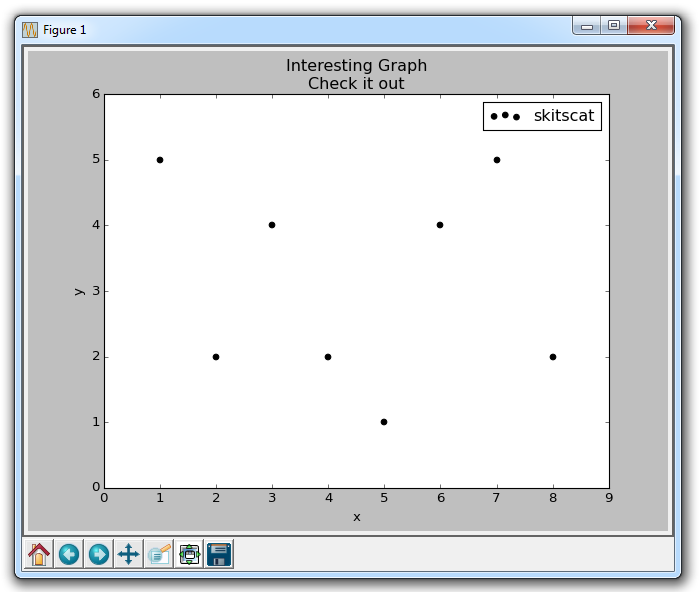# 第四章 散点图

``````import matplotlib.pyplot as plt

x = [1,2,3,4,5,6,7,8]
y = [5,2,4,2,1,4,5,2]

plt.scatter(x,y, label='skitscat', color='k', s=25, marker="o")

plt.xlabel('x')
plt.ylabel('y')
plt.title('Interesting Graph\nCheck it out')
plt.legend()
plt.show()
```````plt.scatter`不仅允许我们绘制`x``y`，而且还可以让我们决定所使用的标记颜色，大小和类型。 有一堆标记选项，请参阅 Matplotlib 标记文档中的所有选项。